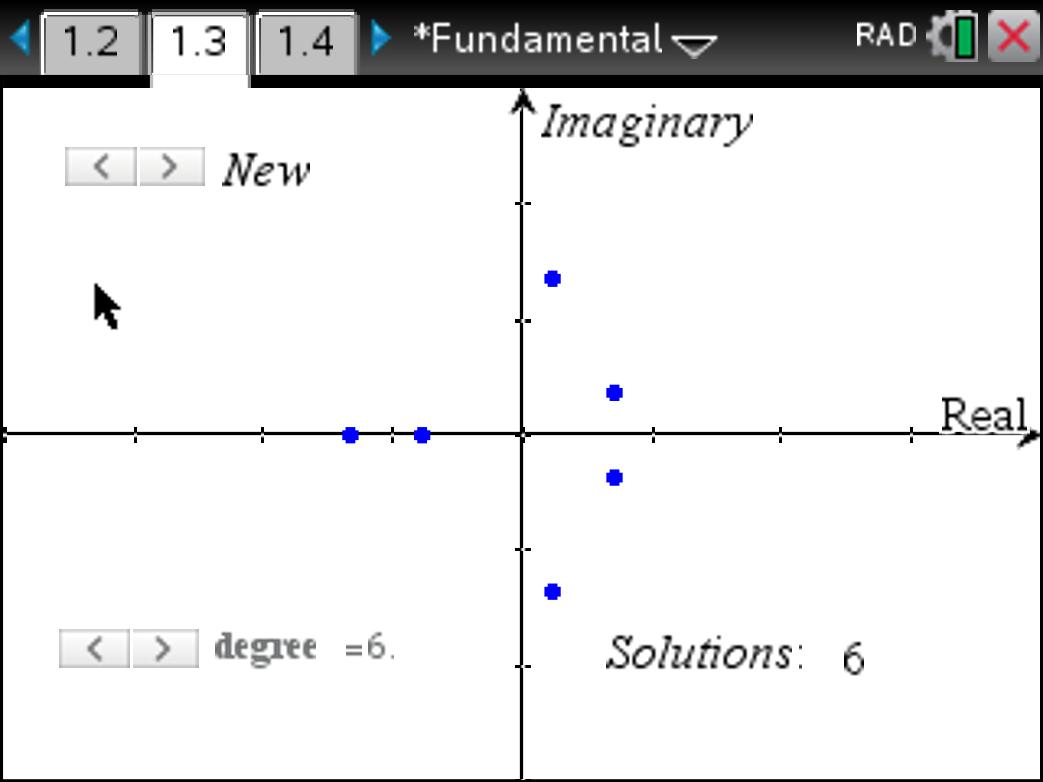Activities

•• Subject Area

• Standard: VCE: Specialist Mathematics: Specialist Mathematics

• AuthorAust Senior

45 Minutes

• Device
• TI-Nspire™ CAS
• TI-Nspire™ Navigator™
• Software

TI-Nspire™ CAS
TI-Nspire™ CAS Navigator™ NC System
TI-Nspire™ Navigator™ NC System

4.0

• Report an Issue

Fundamental Theorem of AlgebraActivity Overview

Students generate random polynomials and observe the distribution of the zeros on the complex plane. From the distributions students are able to draw conclusions about the zeros, and therefore roots, of a polynomial in order to gain a better visual understanding of the Fundamental Theorem of Algebra.

Objectives

Consider conjugate roots for polynomials with real coefficients and the logical extension to understand the Fundamental Theorem of Algebra.

Vocabulary

Polynomial, factor, repeated factor, multiplicity, conjugate, root, zeros